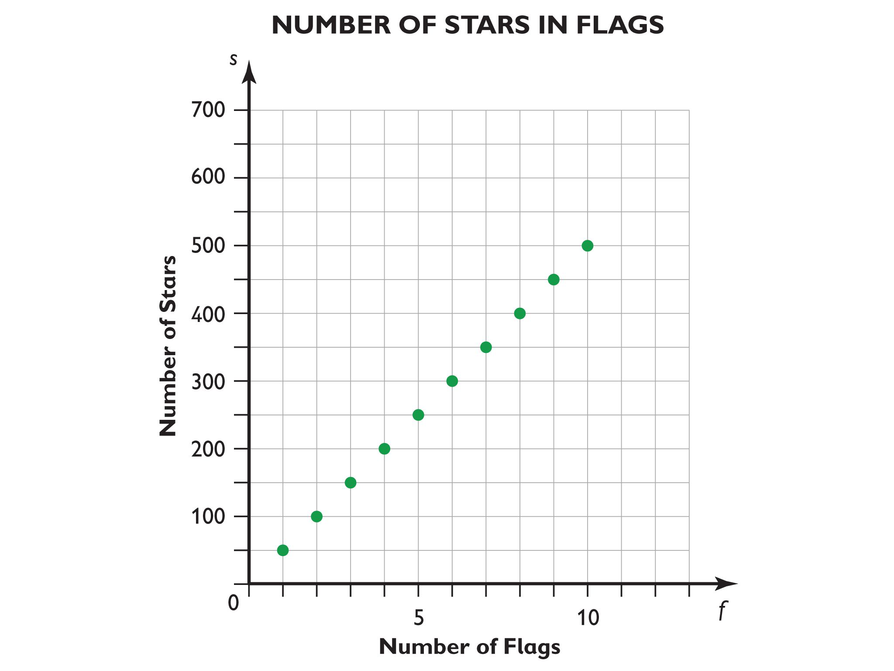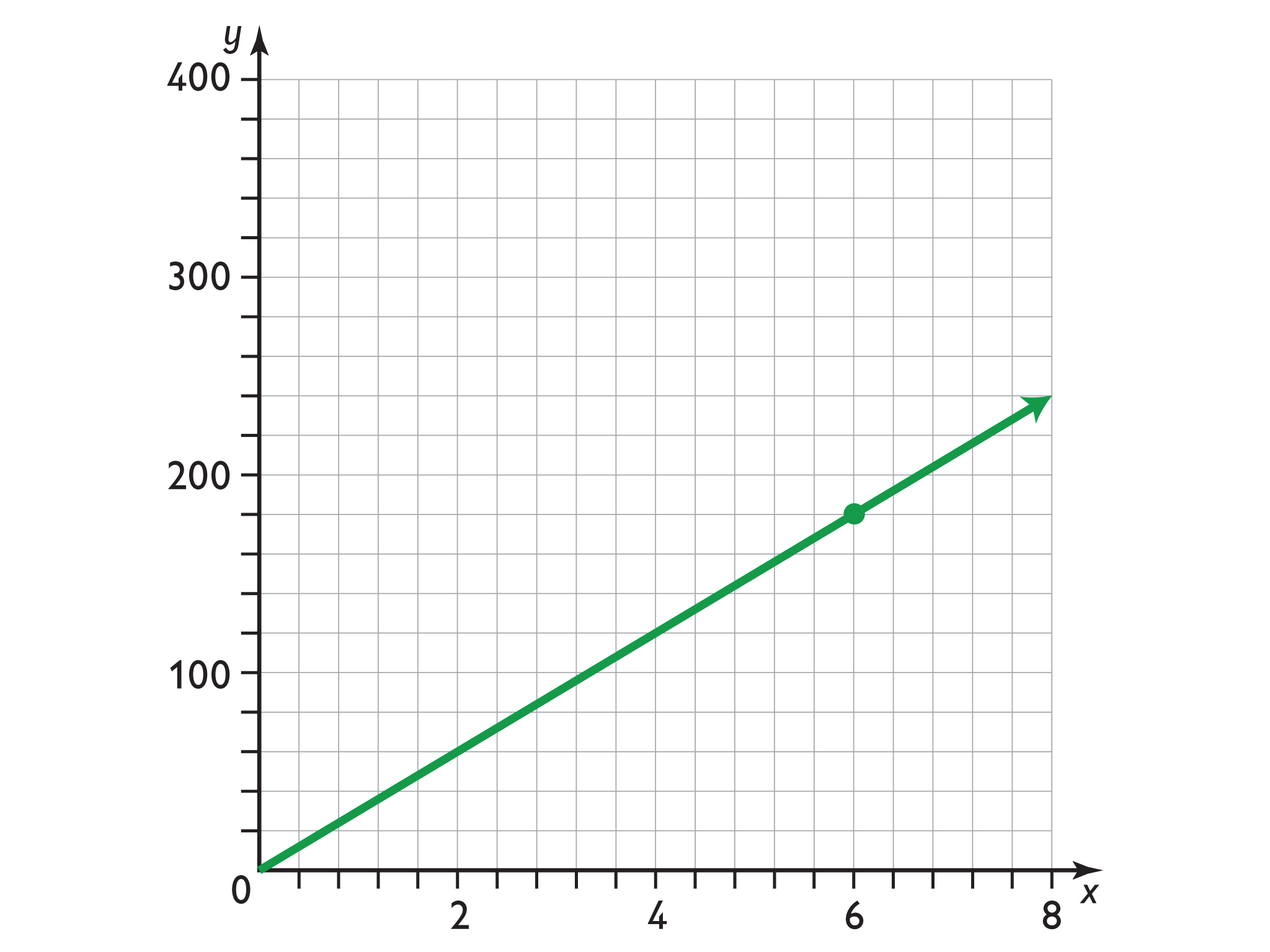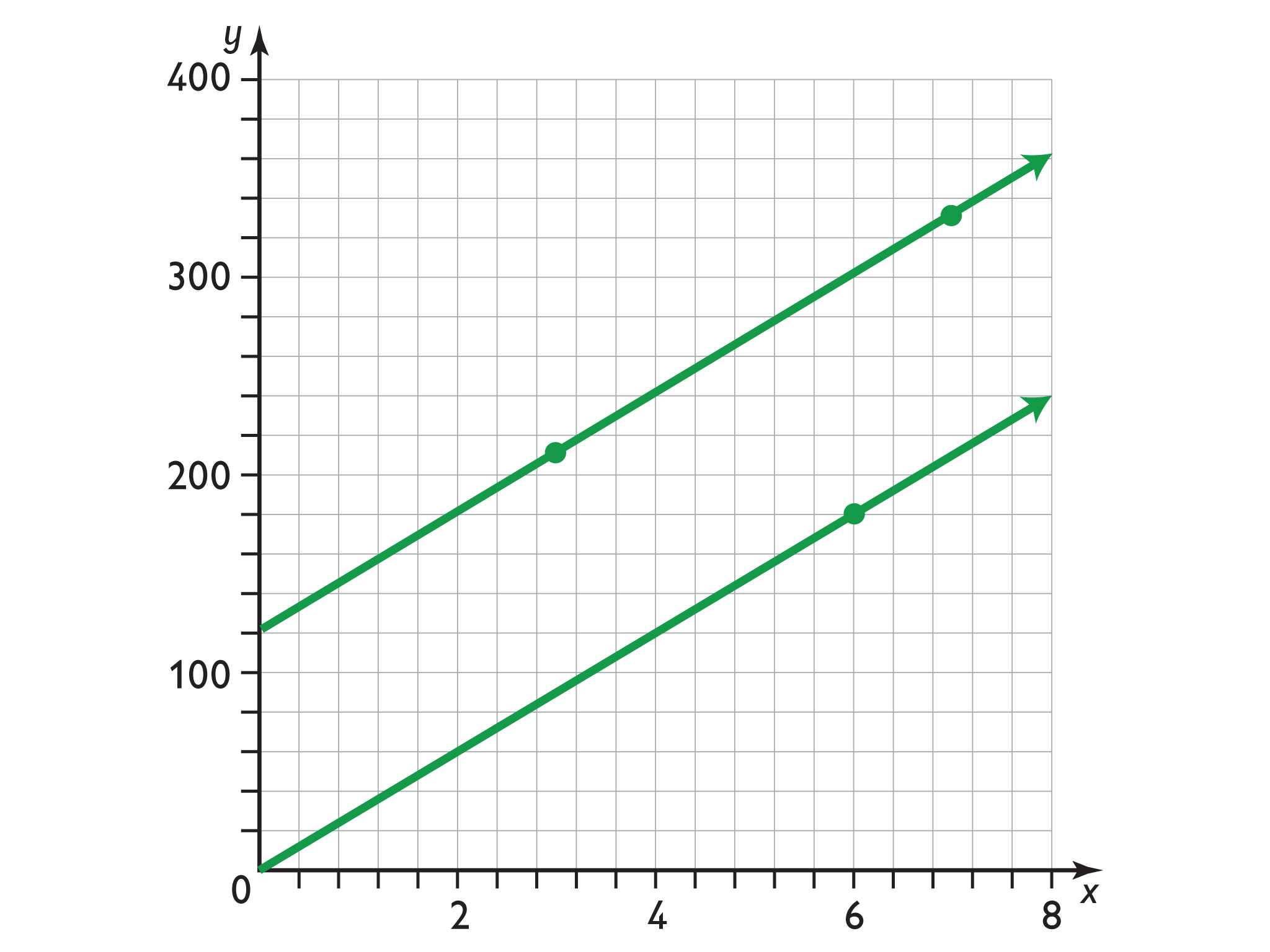Subject:
Ratios and Proportions
Material Type:
Lesson Plan
Level:
Middle School
7
Provider:
Pearson
Tags:
• Proportional Relationship
Language:
English
Media Formats:
Text/HTML

# Formula's Using The Constant Of Proportionality## Overview

Students look at the relationship between the number of flags manufactured and the stars on the flag and determine whether it represents a proportional relationship.

# Key Concepts

• The form of the equation of a proportional relation is y = kx, where k is the constant of proportionality.
• A graph of a proportional relationship is a straight line that passes through the origin.
• The constant of a proportionality in a graph of a proportional relationship is the constant ratio of y to x (the slope of the line).

# Goals and Learning Objectives

• Identify the constant of proportionality in a proportional relationship based on a real-world problem situation.
• Write a formula using the constant of proportionality.
• Analyze a graph of a proportional relationship.
• Make a graph and determine if it represents a proportional relationship.
• Identify the constant of proportionality in a graph of a proportional relationship.

# Lesson Guide

• Have students read the prompt and the two questions posed.

# Mathematics

Students think about the situation described, involving manufacturing flags and preparing the stars for the flags. The two quantities that vary in the proportional relationship are the number of flags to be produced and the number of stars to be prepared for those flags. As students consider the situation, encourage them to think about the different ways of representing the relationship between the quantities (i.e., diagram, table, formula, graph) and how each representation gives evidence that the quantities are or are not proportional (but without actually creating the representations). Ask students:

• What are the quantities in this situation?
• What is one way to know whether or not they are proportional to one another?
• What is another way to know? And another?
• If you graphed the relationship, how would you know (generally) if the quantities are proportional to one another? What would you look for?

Ask the same question about a table, formula, and diagram if you think it would help, but without actually creating these representations.

ELL: Call on ELLs and encourage them to participate, even if their pace is slower or they are reluctant to volunteer due to their weaker command of the language.

• For every flag manufactured, 50 stars need to be prepared. The constant of proportionality is 50.
• The constant of proportionality would be 48.
• Thoughts and discussion will vary.

# U.S. Flags Are US

Jack’s aunt works for a company called U.S. Flags Are US. The company manufactures and sells U.S. flags. Jack’s aunt prepares all the stars.

Sometimes U.S. Flags Are US receives an order for a large quantity of flags. Jack’s aunt wants to determine a rule so she knows how many stars to prepare.

• Explain why the number of stars is proportional to the number of flags. What is the constant of proportionality?
• What would the constant of proportionality have been when the United States consisted of 48 states?

# Lesson Guide

Discuss the Math Mission. Students will apply their knowledge of proportional relationships to solve a real-world problem and make and analyze graphs.

## Opening

Apply your knowledge of proportional relationships to solve a real-world problem and make and analyze graphs.

# Lesson Guide

Have students work in pairs on all problems and the presentation.

# Mathematics

Students focus on graphs of proportional relationships and make a comparison with one graph of a relationship that is linear but not proportional. The graphs involve different scales on the two axes, which presents opportunities to surface certain misconceptions about the coordinate system.

SWD: Students with disabilities may have difficulty graphing information accurately. Provide direct instruction on graphing skills and then allow students to complete the graphs during guided practice. Pay attention to students’ graphs for precision.

ELL: When selecting a group of students to present during Ways of Thinking, ensure students present a topic they are confident about. Have students draw diagrams or demonstrate their knowledge in some other way than through verbal language alone.

• s = 50f

# Making a Flag

• To help Jack’s aunt, write a formula that will tell her how many stars to prepare based on the number of flags ordered.
• Let f represent the number of flags and s represent the total number of stars (50 per flag). Use the constant of proportionality you found to write a formula that represents the relationship between f and s.

## Hint:

The constant of proportionality is the number of stars per flag. What is the rate?

# Lesson Guide

Have students work in pairs on all problems and the presentation.

# Interventions

[common error] Student is confused by the scales on the axes for the graph representing the relationship between f and s.

• Do the scales of the axes have to be the same? Find the point (3, 150) on the graph. What is the meaning of this point in terms of the flags situation?
• Find the point (1, 1) on the graph. What would be the meaning of this point in terms of the flags situation?

• No, the point (206, 1035) does not lie on the graph.

# Point on the Graph

This graph represents the relationship between f and s.

• Does the point (206, 1035) lie on the graph?## Hint:

Could you use your formula to help determine whether the point lies on the graph?

# Lesson Guide

Have students work in pairs on all problems and the presentation.

# Interventions

[common error] Student thinks the points must be connected in the graph representing the relationship between f and s.

• What would the point (5.5, 275) mean in terms of the flags situation?

Student doesn’t sufficiently relate the constant of proportionality with the graphs.

• What is the ratio between the coordinates of each point on the graph?
• Can you make a table to show the points on the graph?
• How is the constant of proportionality illustrated by the graph?• Using y = kx, plug in 180 as the y-value, and 6 as the x-value.

180 = k (6)

$\frac{180}{6}=k$

30 = k

Therefore, the constant of proportionality is 30.

Yes, the graph represents a proportional relationship.

•No, the graph does not represent a proportional relationship. It is a straight line, but it does not intersect the point (0, 0).

The relationship is not proportional, so there is not a constant of proportionality. It's not proportional because the line does not cross through the origin. Therefore the ratio of x to y will be different for two different points on the line.

# More Graphs

• Make a new graph by plotting the points (0, 0) and (6, 180).
Does this graph represent a proportional relationship? If so, what is the constant of proportionality? If not, why not?
• Make another graph on the same coordinate grid by plotting the points (3, 210) and (7, 330). Does this graph represent a proportional relationship? If so, what is the constant of proportionality? If not, explain why it is not a proportional relationship.

## Hint:

How can you tell whether a graph represents a proportional relationship? What must be true about the straight line?

# Preparing for Ways of Thinking

Look and listen for students who:

• Have a clear grasp of the proportional relationship between the number of flags and the number of stars
• Discuss and debate whether 50 is a constant of proportionality
• Discuss the scaling on the axes for the given graph representing the relationship between f and s or for the graphs they must create
• Discuss how the constant of proportionality is shown by the graphs
• Discuss the significance of a graph intersecting the point (0, 0)

# Challenge Problem

• The statement is true. You can draw an abstract graph of a proportional relationship using only a constant of proportionality. But without knowing what the quantities are (and knowing the units for each), it would be hard to draw a graph of their relationship showing values that make sense or are possible (e.g., start and end points, including negative values or not, including non-whole values or not, scaling the axes appropriately, and so on).

# Prepare a Presentation

Prepare a presentation about either the number of stars problem or the two graphs problem. Show and explain all of your work.

# Challenge Problem

When you know the constant of proportionality in a proportional relationship, you have all the information you need to make the graph accurately.

• Is this statement true or false? Explain your thinking.

# Lesson Guide

Elicit student thinking about the graphs of proportional relationships, and provide students opportunities to revise their thinking as needed. Be sure that the discussion includes presentations in which students show the two graphs they created. Have students share their observations about the two graphs. Ask students:

• What do you notice about the two graphs?
• What would happen if you extended the graph in part b so it intersects the y-axis? Where would the line intersect the y-axis? (Have a student do this just by drawing the line out from the existing graph, not by computing values.)
• Both are straight lines, and the lines are parallel. (Build on ideas elicited from students rather than presenting these directly.) So what makes one relationship proportional and the other not?
• What can you say about the values of the coordinates of the points on each graph? Is there a constant ratio?

Close the discussion with presentations of student work on the Challenge Problem. Discuss any differences in thinking to reach a tentative agreement about whether the statement is true or false.

# Ways of Thinking: Make Connections

Take notes about your classmates’ explanations of their work on the number of stars or two graphs problem.

## Hint:

• What is the formula for this situation?
• What would a graph that shows the relationship of the number of stars to the number of flags look like?
• What are the variables?
• What is the constant of proportionality?
• How do you know that the graph represents a proportional relationship?

# A Possible Summary

A graph of a proportional relationship is a straight line that intersects the point (0, 0), meaning when one quantity has a value of 0, the other must too. This is because the ratio between a pair of proportional quantities has a constant value at every point, and if they aren’t 0 at the same time, the ratios wouldn't be proportional at every point.

Discuss the following point as well:

• The form of the formula for a proportional relations is y = kx, where k is the constant of proportionality.

SWD: Make sure all students understand proportional relationships. Have students discuss their understanding with a partner. Have them share in their own words. This will better prepare students for their reflection.

# Summary of the Math: Proportional Relationships

Write a summary about the mathematics of proportional relationships that you used in this lesson.

## Hint:

• Do you provide the form of the formula for a proportional relationship? Do you explain each part of the formula?
• Do you describe the graph of a proportional relationship?